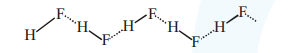# Given below are two statements : one is labelled as Assertion A and the other is labelled as Reason R`
Question:

Given below are two statements : one is labelled as Assertion A and the other is labelled as Reason R

Assertion A : Dipole-dipole interactions are the only non-covalent interactions, resulting in hydrogen bond formation.

Reason $\mathbf{R}$ : Fluorine is the most electronegative element and hydrogen bonds in HF are symmetrical.

In the light of the above statements, choose the most appropriate answer from the options given below.

1. $\mathrm{A}$ is false but $\mathrm{R}$ is true

2. Both $\mathrm{A}$ and $\mathrm{R}$ are true and $\mathrm{R}$ is the correct explanation of $\mathrm{A}$

3. $\mathrm{A}$ is true $\mathrm{R}$ is false

4. Both A and R are true but R is NOT the correct explanation of $\mathrm{A}$

Correct Option: 1

Solution:

Assertion is incorrect since in hydrogen bonding, Dipole-dipole interactions are noncovalent but ion-dipole interaction can also result in H-bond formation. Reason is correct since $F$ is most electronegative element \& structure isSymmetrical H-bonds are present